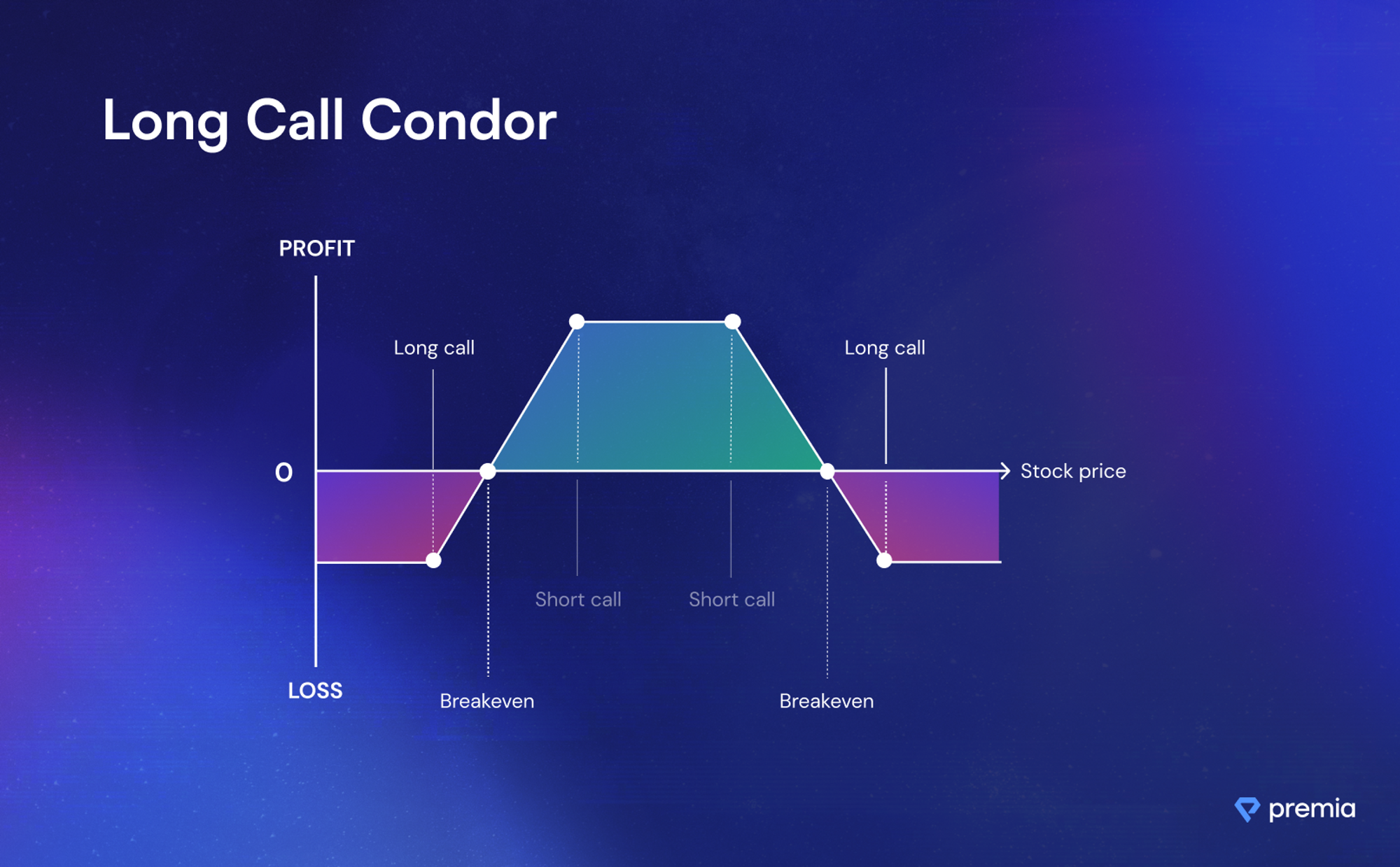## Join The Blue Season on Galxe# Long Call Condor

##### 💡What is a Long Call Condor Spread?

A call condor spread is an options trading strategy that involves combining two vertical spreads (a long call spread and a short call spread) on the same underlying asset with the same expiration date but different strike prices. This strategy is employed when traders anticipate the underlying asset's price to remain within a specific range.

### How do Long Call Condor Spreads Work?

Setting Up the Spread: In a call condor spread, the trader simultaneously establishes a long call spread and a short call spread. This involves buying a call option and selling a further out-of-the-money (OTM) call option (long call spread), while also selling a call option and buying a further OTM call option (short call spread).

Payoff Structure: The payoff structure of a call condor spread is characterized by limited risk and reward, forming a profit range between the two spreads.

### Payoff Structure of a Call Condor Spread

The payoff of a call condor spread depends on the price of the underlying asset at expiration. Here's how it works:

Maximum Gain: The maximum gain is achieved when the underlying asset's price at expiration is between the two strike prices of the short call spread. At this point, the profit potential is at its highest, and it is calculated as the difference between the strike prices of the short call spread minus the net debit paid when setting up the spread.

Maximum Loss: The maximum loss occurs when the underlying asset's price at expiration is outside the range of the long call spread or the short call spread. The loss is limited to the initial debit paid.

Breakeven Points: In a call condor spread, there are two breakeven points. The upper breakeven point is the strike price of the highest-strike short call plus the net debit paid. The lower breakeven point is the strike price of the lowest-strike long call minus the net debit paid.Long Call Condor Payoff Graph

### Considerations for Long Call Condor Spreads

• Range-Bound Market: Call condor spreads are most effective in a range-bound market where the underlying asset's price is expected to remain within a specific range. The strategy benefits from time decay and limited price movements.
• Limited Profit Potential: Call condor spreads have a limited profit potential, as the underlying asset's price must remain within a specific range for optimal results. Traders should assess whether the potential profit justifies the costs and risks involved.
• Risk Management: It's crucial to set appropriate risk management parameters, including defining the maximum loss and adjusting or closing the position if the underlying asset's price starts moving significantly beyond the range of the spreads.
• Time Decay: Call condor spreads benefit from time decay, meaning the value of the options may decrease as expiration approaches.
###### Test Yourself!

What does the Long Call Condor consist of?

• A combination of two vertical spreads (a long call spread and a short call spread) on the same underlying asset with the same expiration date but different strike prices.

• A combination of two debit spreads on two different underlying assets.

• A combination of three vertical spreads on the same underlying asset with the same expiration date but different strike prices.